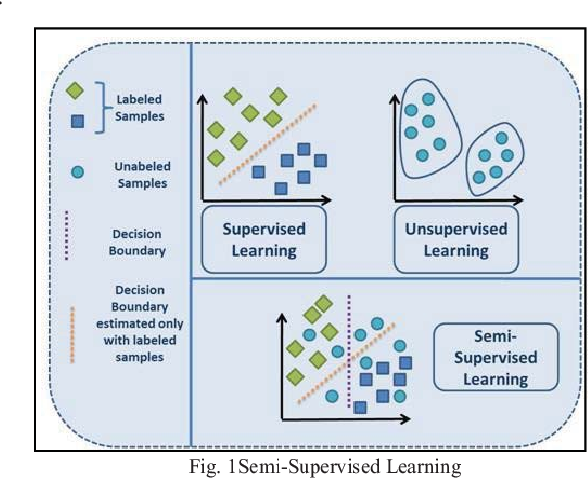Home Machine Learning Machine Learning Education Understanding Pseudo Labelling

# Understanding Pseudo Labelling

 Audio version of the article

There are 3 kinds of machine learning approaches- Supervised, Unsupervised, and Reinforcement Learning techniques. Supervised learning as we know is where data and labels are present. Unsupervised Learning is where only data and no labels are present. Reinforcement learning is where the agents learn from the actions taken to generate rewards.

Imagine a situation where for training there is less number of labelled data and more unlabelled data. A new technique called Semi-Supervised Learning(SSL) which is a mixture of both supervised and unsupervised learning. As the name suggests, semi-supervised learning has a set of training data which is labelled and another set of training data, which is unlabelled. We can think of this situation as when Google photos or Facebook identify people in the picture by their faces(data) and generate a suggested name(label) based on the previously stored images of that person.

Source – LinkIn this article, I’ll be discussing how to generate pseudo labels using the semi-supervised learning technique.

Pseudo-Labelling

Pseudo labelling is the process of using the labelled data model to predict labels for unlabelled data. Here at first, a model has trained with the dataset containing labels and that model is used to generate pseudo labels for the unlabelled dataset. Finally, both the datasets and labels(original labels and pseudo labels) are combined for a final model training. It is called pseudo(which means unreal) as these may or may not be real labels and we are generating them based on a similar data model.

Source – Link

Implementation in Python

For this demonstration, I’ve taken up the sklearn dataset breast cancer. I know that it already contains labels but we are going to modify it by splitting the data into two parts one having labels and the other with no labels. We’ll generate our own labels for the unlabelled data from the labelled data model that has been trained and then finally use both to train a final model.

Dataset:

Breast cancer dataset is a classification problem to predict whether the cancer is benign(B) or malignant(M). First two columns being 1)id and 2)diagnosis(target)

Feature set contains :

a) radius_mean (mean of distances from the centre to points on the perimeter)

b) texture_mean (standard deviation of gray-scale values)

c) perimeter_mean

d) area_mean

e) smoothness_mean (local variation in radius lengths)

f) compactness_mean (perimeter^2 / area – 1.0)

g) concavity_mean (severity of concave portions of the contour)

h) concave points_mean (number of concave portions of the contour)

Importing libraries

```import pandas as pd
import numpy as np
from sklearn.model_selection import train_test_split
from sklearn.datasets import load_breast_cancer
from sklearn.ensemble import RandomForestClassifier```

Loading dataset

```X,y = load_breast_cancer(True)
X.shape```

(569, 30)

Splitting dataset into data with labels and data with no labels in 40:60 ratio

```x_train,x_test,y_train,_ = train_test_split(X,y,test_size=.6)
x_train.shape,y_train.shape,x_test.shape```

((227, 30), (227,), (342, 30)

Model Creation and fitting the data containing labels

```model1 = RandomForestClassifier()
history = model1.fit(x_train,y_train)
history```

RandomForestRegressor(bootstrap=True, ccp_alpha=0.0, criterion=’mse’,

max_depth=None, max_features=’auto’, max_leaf_nodes=None,

max_samples=None, min_impurity_decrease=0.0,

min_impurity_split=None, min_samples_leaf=1,

min_samples_split=2, min_weight_fraction_leaf=0.0,

n_estimators=100, n_jobs=None, oob_score=False,

random_state=None, verbose=0, warm_start=False)

Accuracy score for data-label model training

`model1.score(x_train,y_train)`

1.0

Now we use this model to predict labels (called pseudo labels) for no label data

```y_new = model1.predict(x_test)
y_new.shape```

(342,)

We concatenate both these datasets now

```final_X = np.concatenate((x_train,x_test))
final_X.shape```

(569, 30)

Similarly both labels(original and pseudo) are also concatenated.

```final_Y = np.concatenate((y_train,y_test))
final_Y.shape```

(569,)

Final model containing entire dataset is fitted and accuracy score is generated

```model2 = RandomForestRegressor()
model2.fit(final_X,final_Y)
model2.score(final_X,final_Y)```

1.0

Conclusion

This was the implementation of pseudo labelling. I’ve modified an existing dataset with labels but it can similarly be used in real-world data science scenarios to achieve pseudo labels for unlabelled data from labelled data. Semi-supervised learning has now gained much attention in both classical machine learning problems as well as deep learning.

This article has been published from the source link without modifications to the text. Only the headline has been changed.

Source link

- Advertisment -### Lockdowns’ benefit on Data Centre buildings

- Advertisment -